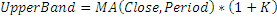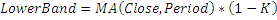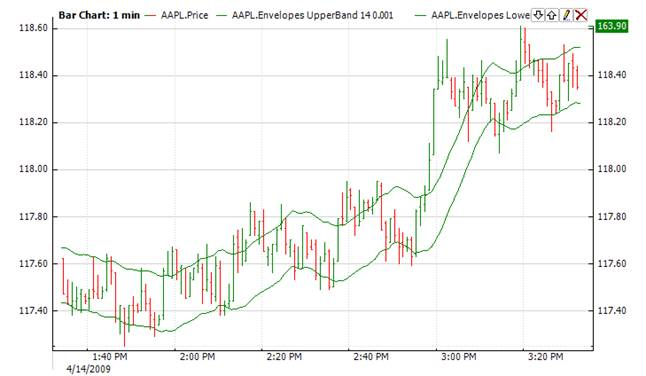﻿Envelope

# Envelope

Envelope technical Indicator is formed with two moving averages; one is shifted upward and the other is shifted downward.  The selection of optimum relative number of band margins shifting is determined with market volatility: the higher the volatility, the stronger the shift.Market Signals

Envelope defines the upper and the lower margins of the price range. When the price reaches the upper margin of the band, the indicator creates a sell signal. When the price reaches the lower margin of the band, the indicator creates a buy signal.CalculationChart ExampleImplementation and Usage

To initialize Envelope indicator use one of the following constructors:

Envelope – sets default values: period = 14, factor = 0.001 (K in the formula)

Envelope(Int32, Double) – sets values for period and factor

Envelope(TimeSpan, Double) – sets time period and factor

Envelope(IAverager, Double) – sets type of averaging and factor

Use

UpperBand

LowerBand

properties to get current values.Example
C#
``` 1// Create new instance
2Envelope env = new Envelope();
3
4// Number of stored values
5env.HistoryCapacity = 2;
6
9
10// Get indicator value
11double IndUpperBand = env.UpperBand;
12double IndLowerBand = env.LowerBand;
13// Get previous value
14if (env.HistoryCount == 2)
15{
16    double IndicatorPrevValue = env;
17}```

Envelope with custom averaging:

C#
```1// Create new instance, but instead SMA we will use EMA, period = 14
2Envelope env = new Envelope(new Ema(14), 0.001);
3